Adding vectors results in a new Resultant Vector. Geometric and algebraic vectors can be added using different techniques.

Geometric vectors can be added by positioning the vectors head to tail or tail to tail. Remember, you can move a vector around in space as long as it maintains its magnitude and direction.

To add vectors head to tail, move one vector so that it's tail starts at the head of the previous vector. Repeat for all vectors being added teogether. The Resultant Vector starts at the tail of the first vector and ends at the head of the last one.

Below are the steps to add vectors $$\vec{u}$$ and $$\vec{v}$$ head to tail. Sometimes, this method is referred to the Triangle Law of Vector Addition.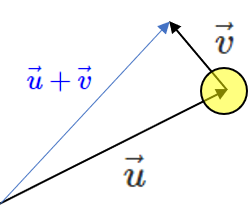These steps can be extended to more than one vector.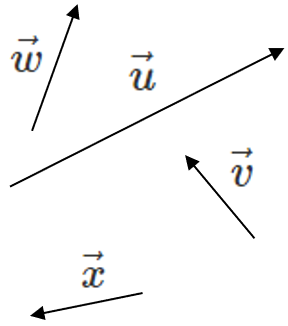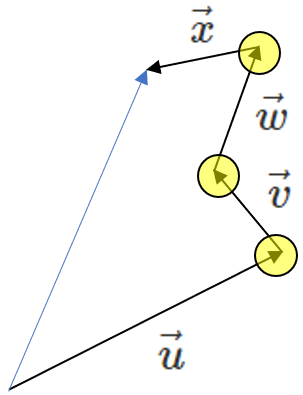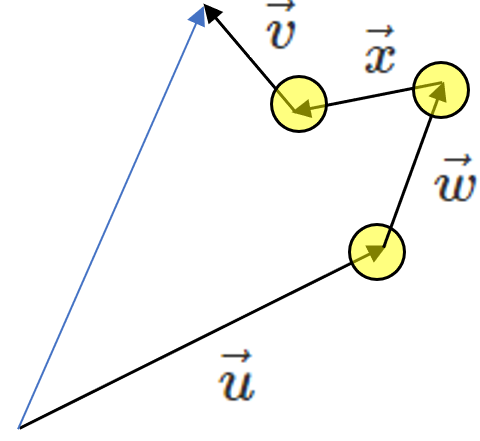Notice that it doesn't matter which order you add the vectors in. These rules are shown below:

 Commutative Law of Addition $$\vec{u} + \vec{v} = \vec{v} + \vec{u}$$ Associative Law of Addition $$(\vec{m} + \vec{n}) + \vec{o} = \vec{m} + (\vec{n} + \vec{o})$$

Adding geometric vectors involves solving triangles using trigonometry equations. Below is a summary:

 Right Triangles Pythagorean Theorem $$c^2 = a^2 + b^2$$ SOH CAH TOA $$sin(\theta) = \frac{opp}{hyp} = \frac{y}{r}$$ $$cos(\theta) = \frac{adj}{hyp} = \frac{x}{r}$$ $$tan(\theta) = \frac{opp}{adj} = \frac{y}{x}$$
 Other Triangles Cosine Law $$c^2 = a^2 + b^2 - 2 a b cos(\theta)$$ Sin Law $$\frac{sin(A)}{a} = \frac{sin(B)}{b} = \frac{sin(C)}{c}$$

While driving, Zaina travels $$5 \; [km] \; N$$ and then $$2 \; [km] \; E$$. Find her displacement.

##### Try these questions:
Frank and Michelle take their son Marco tobogganing. On the way to the slopes, Macro sits on the toboggan and Frank and Michelle each pull on a string attached to the front. Frank applies a force of 45 [N] $$30^\circ$$ away from the toboggan. Michelle applies a force of 26 [N] $$60^\circ$$ away from the toboggan in the other direction. What is the resultant force applied to Marco on the toboggan?

Frank and Michelle take their son Marco tobogganing. On the way to the slopes, Macro sits on the toboggan and Frank and Michelle each pull on a string attached to the front. Frank applies a force of 45 [N] $$30^\circ$$ away from the toboggan. Michelle applies a force of 26 [N] $$30^\circ$$ away from the toboggan in the other direction. What is the resultant force applied to Marco on the toboggan?

Supose you add $$\vec{AB} + \vec{BC}$$. Adding the vectors head to tail shows that the result is $$\vec{AC}$$. When the middle letters match, you can "collapse" them to quickly find the Resultant Vector.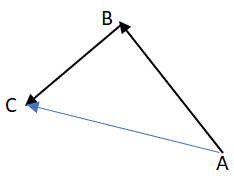In pentagon ABCDE, what is $$\vec{AB} + \vec{DE} + \vec{BD}$$?